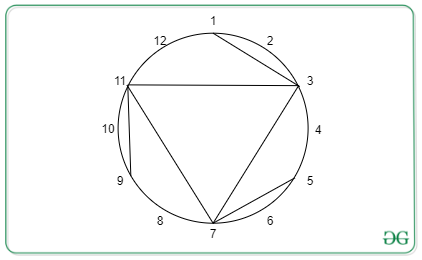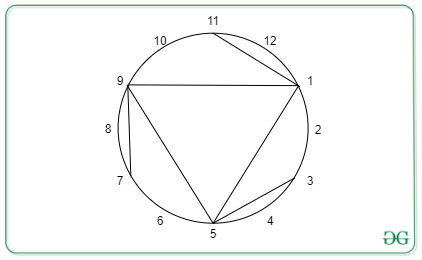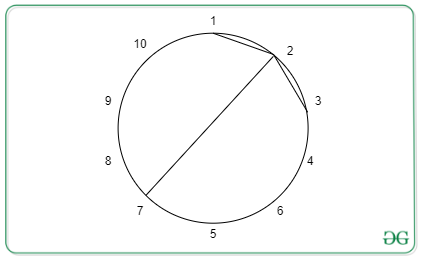# Check if chords of a Circle are symmetric after some rotation

• Difficulty Level : Hard
• Last Updated : 21 Aug, 2021

Given two Integers N and M, N indicating equidistant points on circumference of a circle and M indicating number of chords formed with those points. Also given is a vector of pairs C containing position of chords. The task is to rotate the circle by any degree, say X, where 0 < X < 360, and check if the chords of are still symmetric to the original circle.

Example:

Input: N = 12, M = 6, C = {{1, 3}, {3, 7}, {5, 7}, {7, 11}, {9, 11}, {11, 3}};
Output: YESOriginalAfter Rotation

Input: N = 10, M = 3,  C = {{1, 2}, {3, 2}, {7, 2}}
Output: NONo rotational symmetry possible

Naive Approach: Rotate for every distance K in the range[1, N] and check for each point [a, b] if the rotated point [a + K, b + K] exits.
If there exists any k then print YES else print NO
Time Complexity: O(N*M)

Efficient Approach: It is enough to check for the divisors of N
Let us suppose if we rotate the image by K units then the whole image will be divided into N/K blocks. Then if K is not a divisor of N, there will be an asymmetric block of length less than K and the image will never be symmetric to the original figure.
So calculate all the divisors of N and check for each chord the rotated chord exists or not.

Below is the implementation of the above approach:

## C++

 `// C++ Program to for the above approach` `#include ``using` `namespace` `std;` `// Utility function to calculate``// divisors of a number in O(sqrt(N))``vector<``int``> calculateDivisors(``int` `N)``{``    ``vector<``int``> ``div``;``    ``for` `(``int` `i = 1; i * i <= N; i++) {``        ``if` `(N % i == 0) {``            ``div``.push_back(i);``            ``if` `(N / i != i && i != 1) {``                ``div``.push_back(N / i);``            ``}``        ``}``    ``}``    ``return` `div``;``}``int` `checkRotationallySymmetric(``    ``vector > A,``    ``int` `N, ``int` `M)``{``    ``// Maintain a set to check quickly``    ``// the presence of a chord``    ``set > st;``    ``for` `(``int` `i = 0; i < M; i++) {``        ``--A[i].first, --A[i].second;``        ``if` `(A[i].first > A[i].second) {``            ``swap(A[i].first, A[i].second);``        ``}``        ``st.insert(A[i]);``    ``}` `    ``// Calculate the divisors of N.``    ``vector<``int``> ``div` `= calculateDivisors(N);` `    ``// Iterate through the divisors``    ``for` `(``auto` `x : ``div``) {``        ``bool` `exist = 1;``        ``for` `(``int` `i = 0; i < M; i++) {``            ``int` `dx = (A[i].first + x) % N;``            ``int` `dy = (A[i].second + x) % N;``            ``if` `(dx > dy) {``                ``swap(dx, dy);``            ``}``            ``if` `(st.find({ dx, dy }) != st.end()) {` `                ``// There exists a valid``                ``// chord after rotation``            ``}``            ``else` `{` `                ``// There is no valid chord after rotation``                ``exist = ``false``;``                ``break``;``            ``}``        ``}` `        ``// if there exist another chord after``        ``// rotation for every other chord print``        ``// YES and exit the function``        ``if` `(exist) {``            ``cout << ``"YES"``;``            ``return` `0;``        ``}``    ``}``    ``cout << ``"NO"``;``    ``return` `0;``}` `// Driver Code``int` `main()``{``    ``int` `N = 12, M = 6;``    ``vector > C``        ``= { { 1, 3 }, { 3, 7 }, { 5, 7 },``             ``{ 7, 11 }, { 9, 11 }, { 11, 3 } };``    ``checkRotationallySymmetric(C, N, M);``    ``return` `0;``}`
Output
`YES`

Time Complexity: O(M*sqrt(N)*log M)
Space Complexity: O(M)

My Personal Notes arrow_drop_up